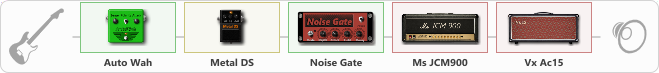# Auto Wah + DS

Discussion in 'ToneLib-GFX presets' started by brunko31, Feb 9, 2020.

1. Auto Wah + DS

Preset name: Auto Wah + DS

Effects chain:Effect: "Auto Wah" (Dynamics / Filter), active - "yes"
{
"Sense" = 50
"Polarity" = Up
"Attack" = 74
"Style" = V847
}

Effect: "Metal DS" (Overdrive / Distortion), active - "yes"
{
"Dist" = 100
"Bass" = 74
"Middle" = 60
"Treble" = 46
"Level" = 75
}

Effect: "Noise Gate" (Dynamics / Filter), active - "yes"
{
"Mode" = Auto
"Depth" = 50
"Threshold" = 46
"Attack" = 172
"Hold" = 5
"Decay" = 250
}

Effect: "Ms JCM900" (Amp simulators), active - "yes"
{
"Gain" = 2
"Bass" = 50
"Middle" = 50
"Treble" = 33
"Presence" = 50
"Master" = 100
"Level (dB)" = 0
}

Effect: "Vx Ac15" (Amp simulators), active - "yes"
{
"Gain" = 50
"Bass" = 0
"Middle" = 0
"Treble" = 50
"Presence" = 0
"Master" = 82
"Level (dB)" = 5
}

Note: You will need to download and install the ToneLib-GFX software to use the preset.

File size:
561 bytes
Views:
3,313